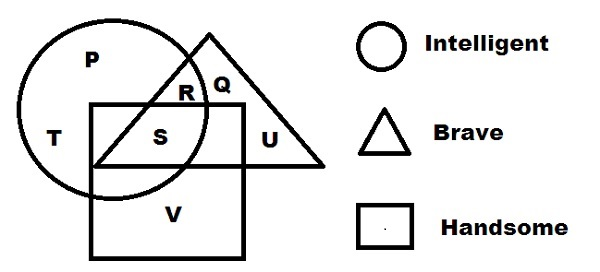# Logical Venn Diagrams - Solved Examples

Study the following diagram and try to answer the question accordingly.Q 1 − Which letter among the following is both intelligent and brave?

Options :

A - P

B - Q

C - R

D - U

Explanation

R lies in the intersection point of both triangle and circle.

Q 2 − Which letter among the following is intelligent but not brave or handsome?

Options :

A - T

B - Q

C - V

D - U

Explanation

T lies in the circle area.

Q 3 − Which letter among the following is intelligent, handsome and brave?

Options :

A - P

B - Q

C - R

D - S

Explanation

S lies in the intersection point of triangle, rectangle and circle.

Explanation

V lies in rectangle area only.

Explanation

P lies only in the circle area.

Explanation

As U lies in triangle area only, hence it is only brave.

Q 7 − Who among the following is brave but neither intelligent nor handsome?

Options :

A - Q

B - R

C - S

D - U

Explanation

Q lies only in the triangle area.

Explanation

Both XP and vista are categories of operating system.

Explanation

Some PDFs may be magazines or French magazine.